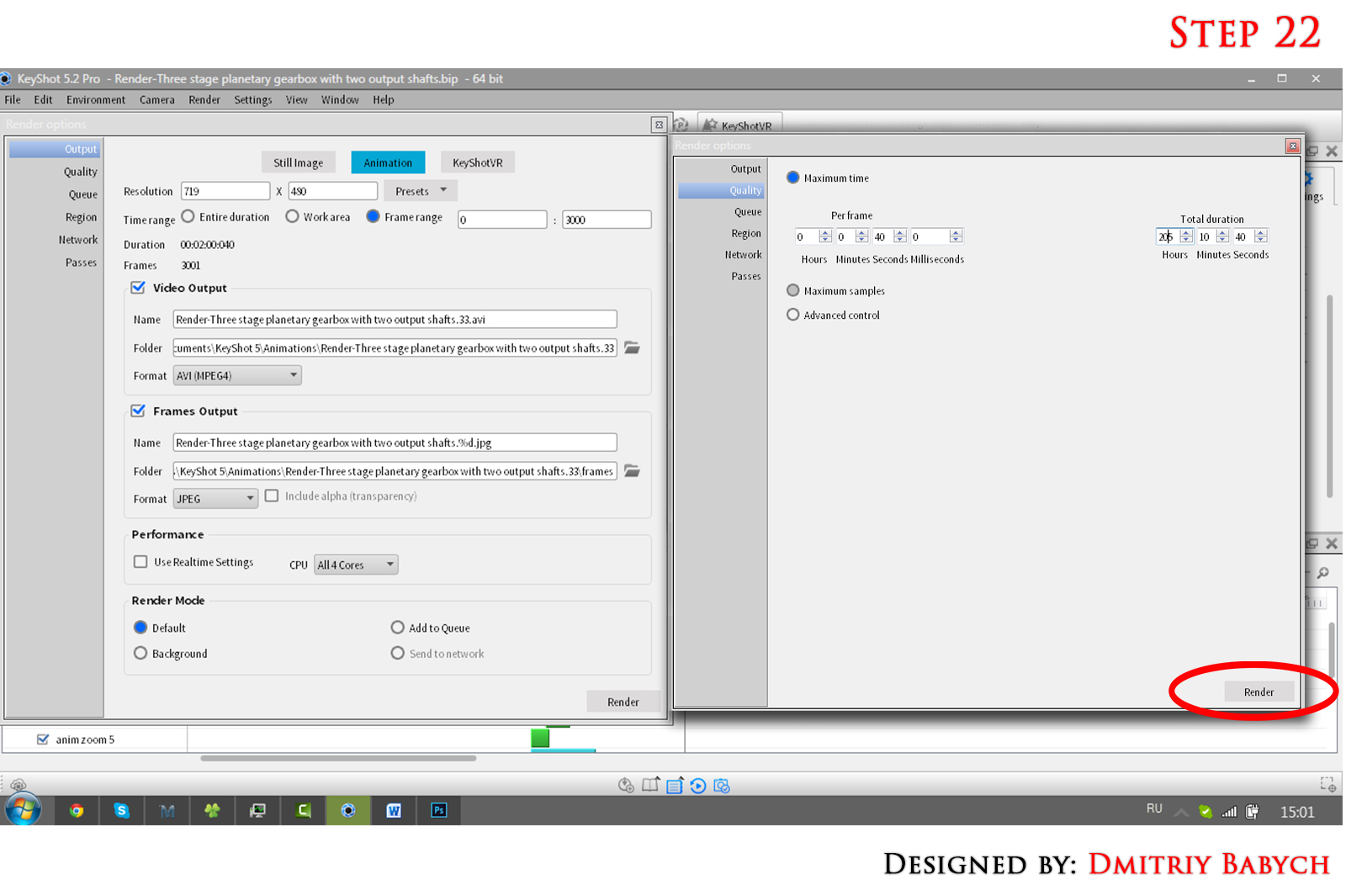# How to animate planetary gearbox in keyshot?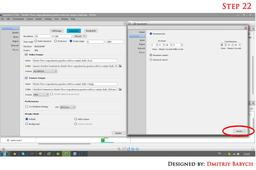Step by step tutorial! Don`t want read, watch video https://youtu.be/p75CQb14X5M

1. ### Step 1:

At first, we will have done some calculations. We have need to know gear ratio, rotation speed in rpm and degree of every element in this gearbox. For calculations I used MatchCAD. It isn't so important to use it program, you can calculate with any software or do it manually. All calculations you can download from this page or from this project.
At first create new MathCAD document. Enter the number of gear teeth of each stage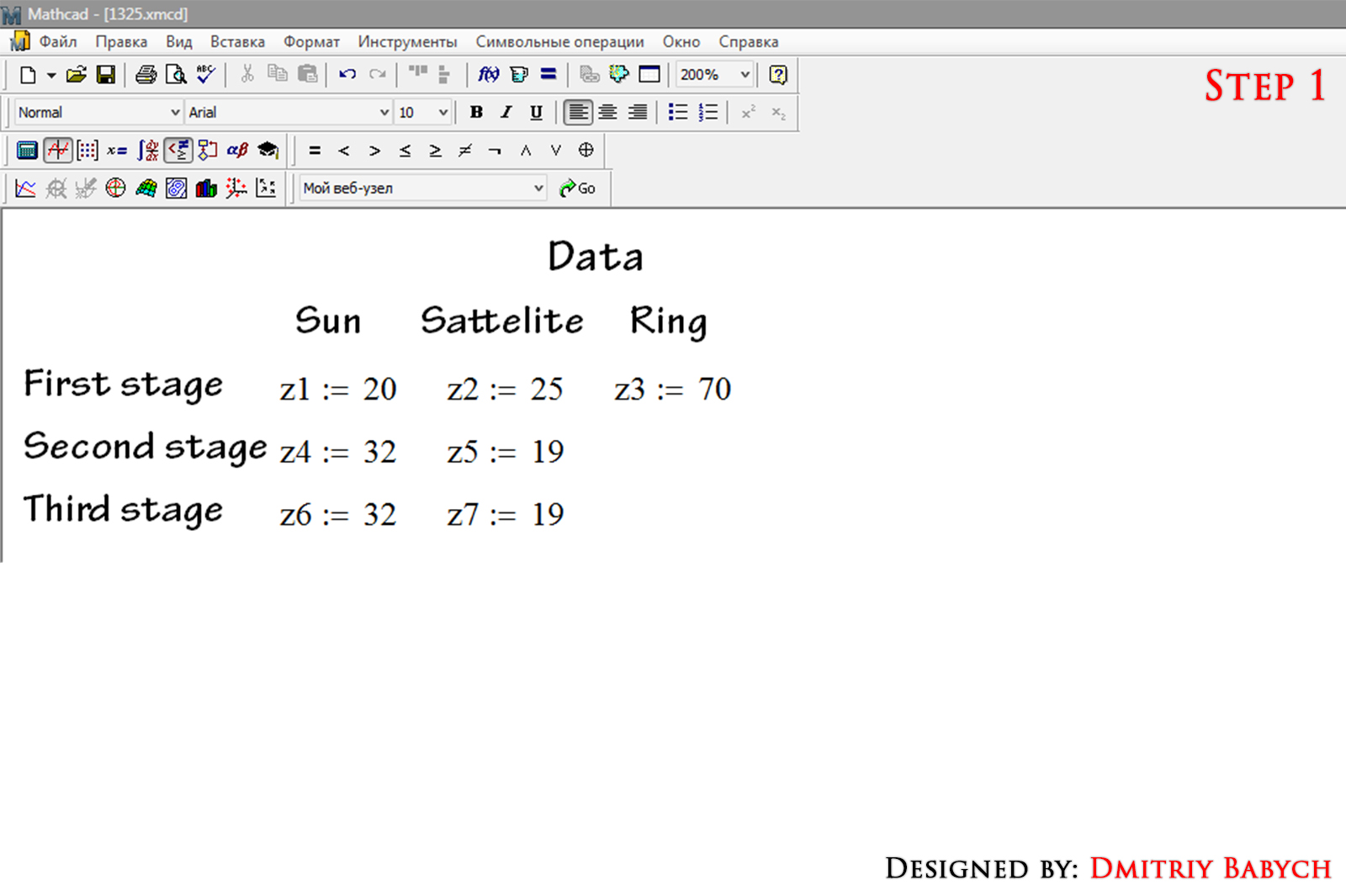2. ### Step 2:

Calculating k - multiplicity of the planetary gear (for every stage)
k= z(ring)/z(sun)
k1=70/25
k2=70/32
k3=70/32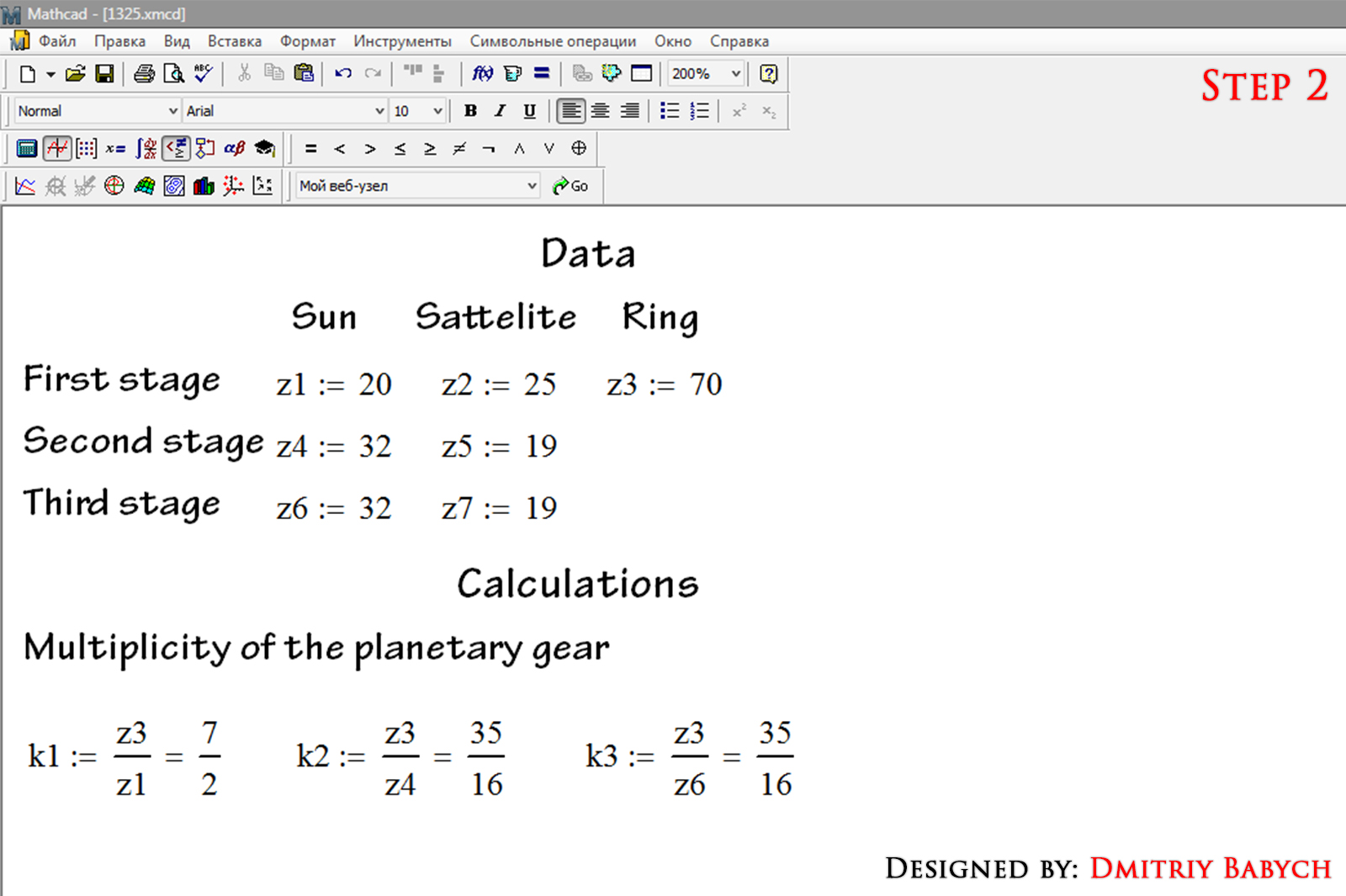3. ### Step 3:

Calculations u – gear ratio (for every stage)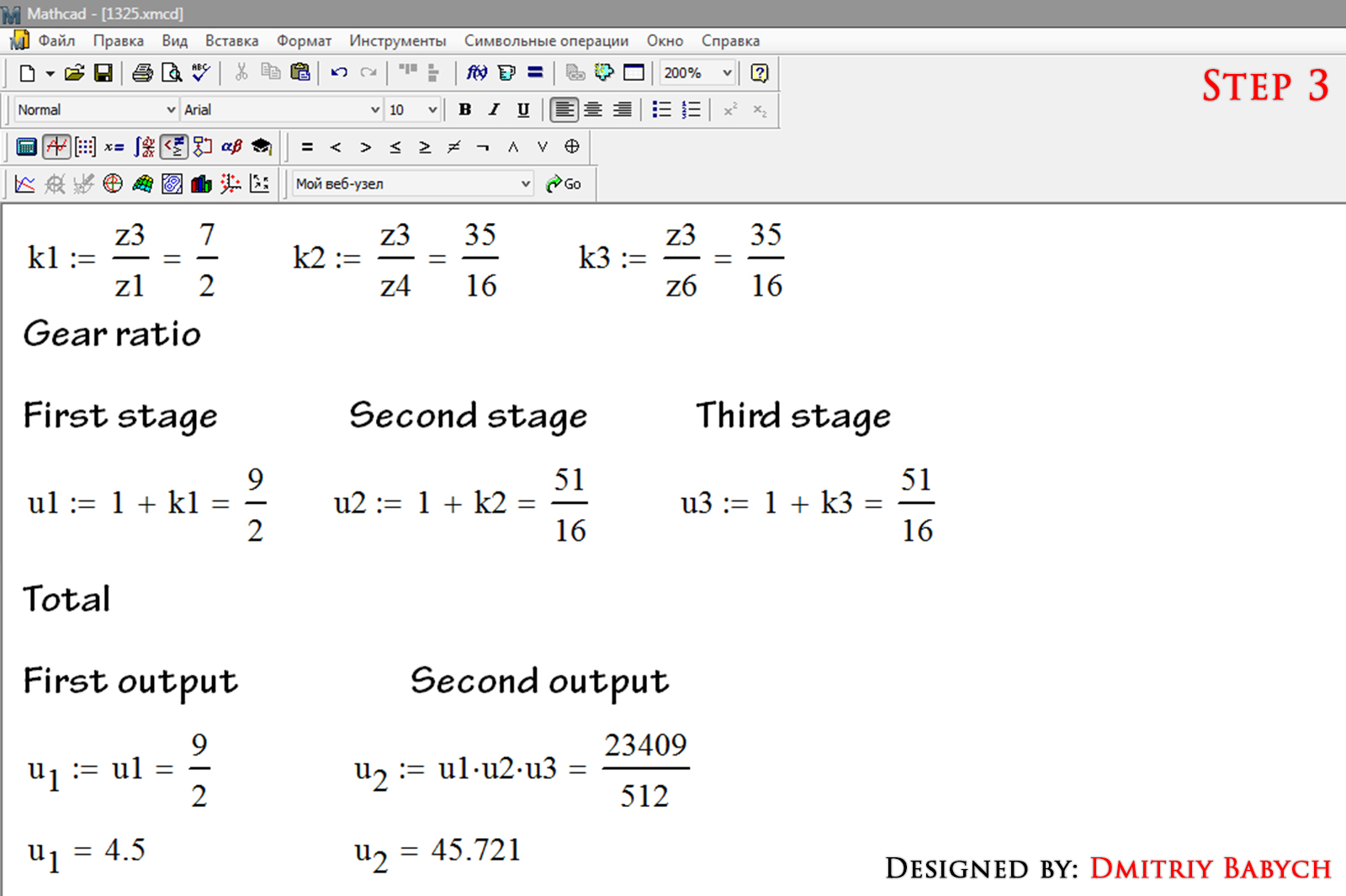4. ### Step 4:

Now calculate rotation of every element of gearbox.

ATTENTION: In Keyshot rotation is written in degrees of rotation angle. The number should contain no more than 6 digits with a decimal coma. You can enter only one decimal number after the coma. This program features.

Now we will calculate the rotation and correcting results what to write it in Keyshot correctly numbers.

First stage
Incoming speed we add manually (then we correct it). Next, will calculate using a formulas. Formulas on the screenshot. “Element ratio to input rotation” will help us in future find correct input speed.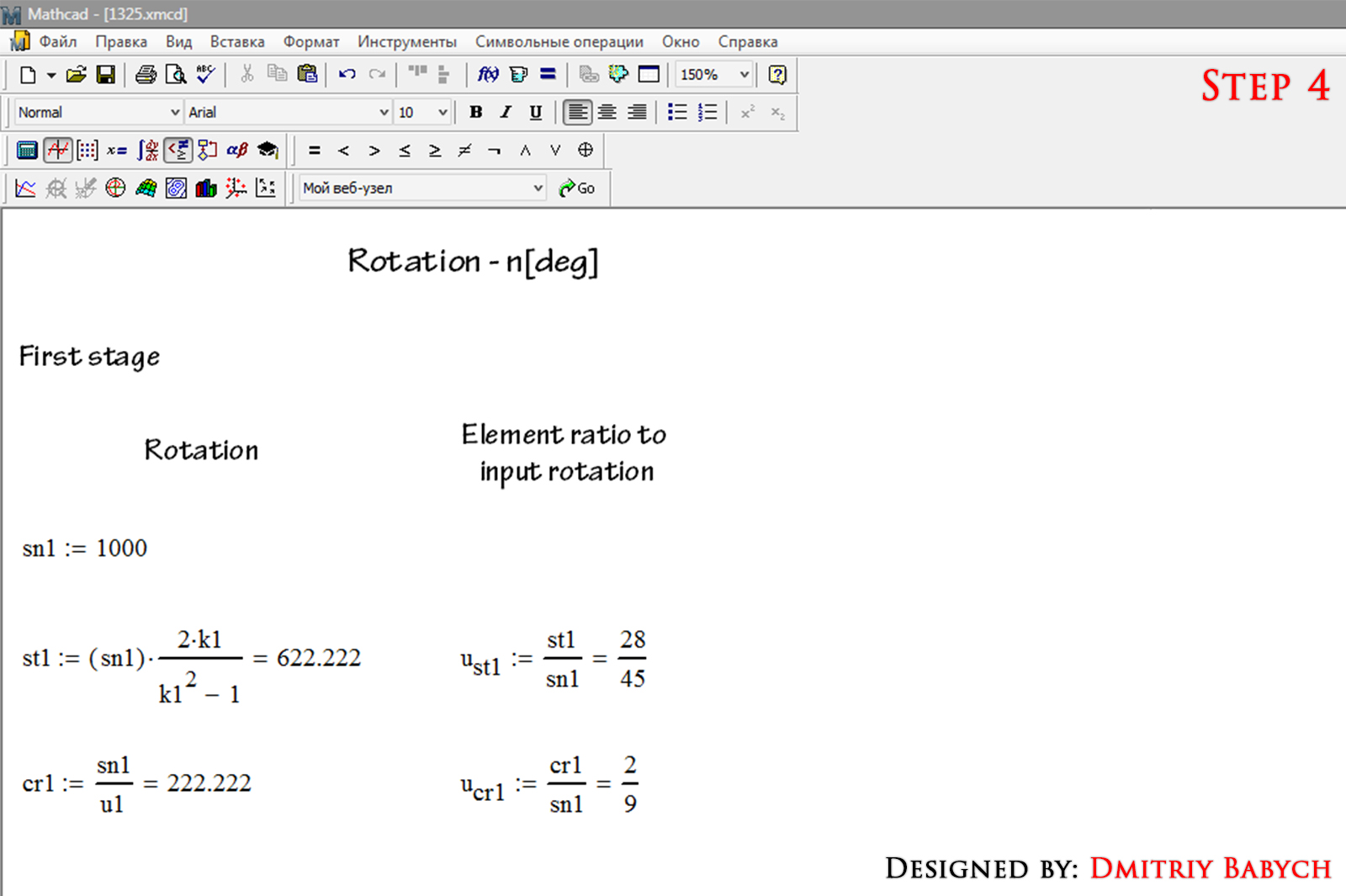5. ### Step 5:

Second stage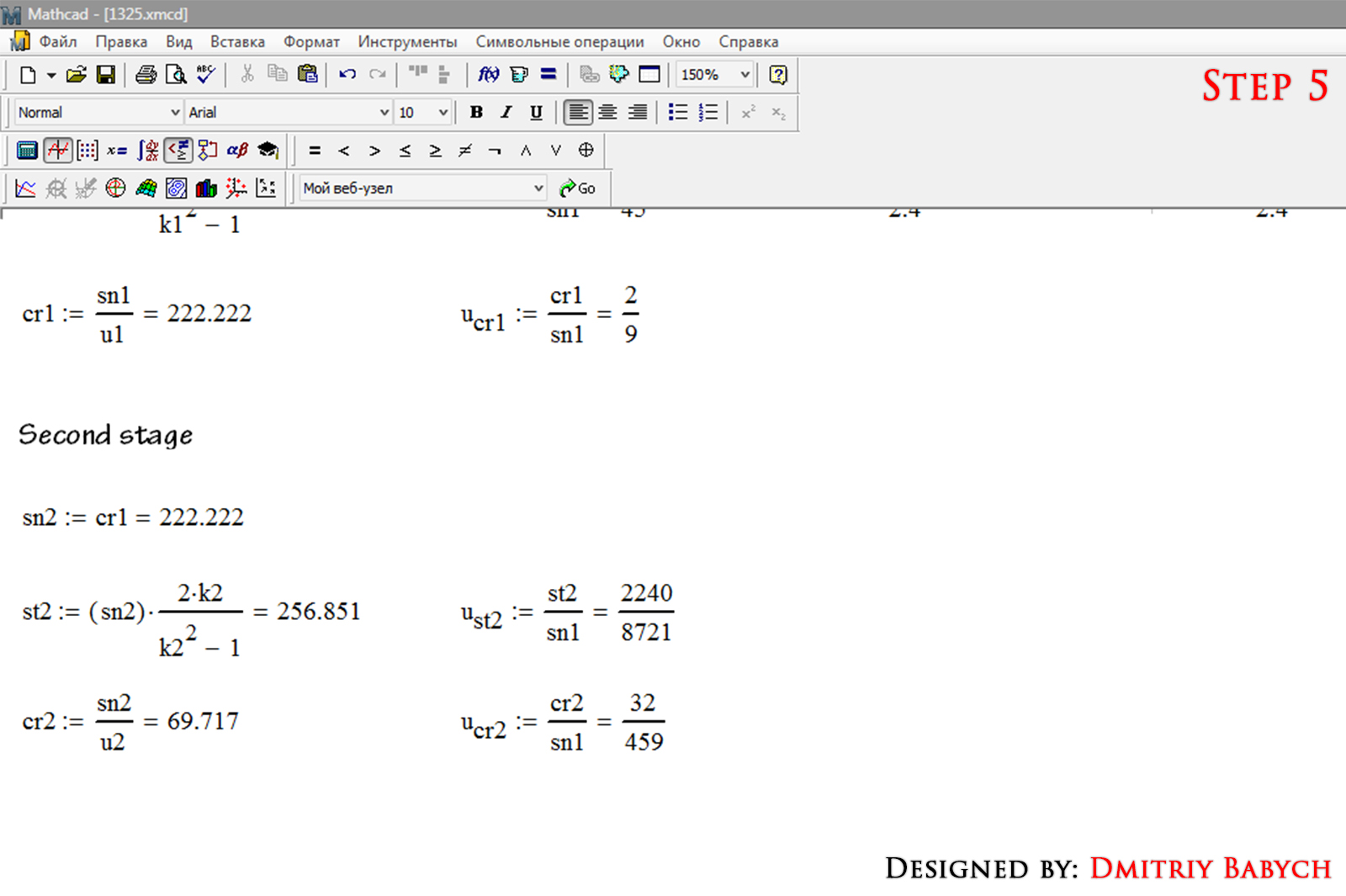6. ### Step 6:

Third stage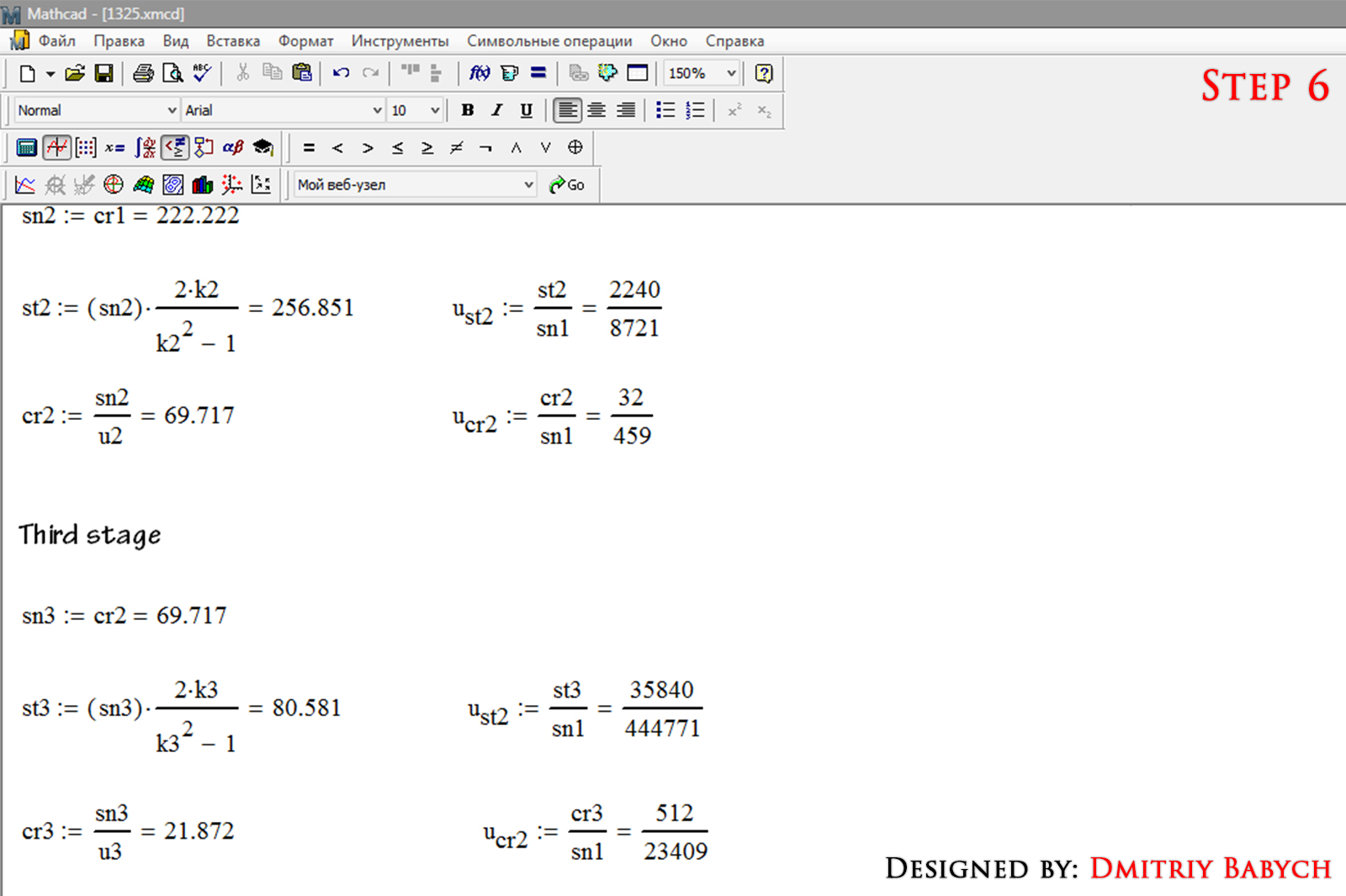7. ### Step 7:

Using “Element ratio to input rotation” correct rotation of all elements, that they would have will meet requirements from step 4. By selecting numbers from denominator, we set starting rotation to 444771 degrees. All the momentum matches the conditions, except satellite from first stage. We will correct it in next step.

In Keyshot we will have to set the rotation of each component. Output shaft rotate to 9728 degrees which corresponds 27,022 turns. Set time of rotation to 12 minutes In Keyshot. In that way, we get 2.252 rpm on output shaft. This is done to scale RPM for more beautiful animation.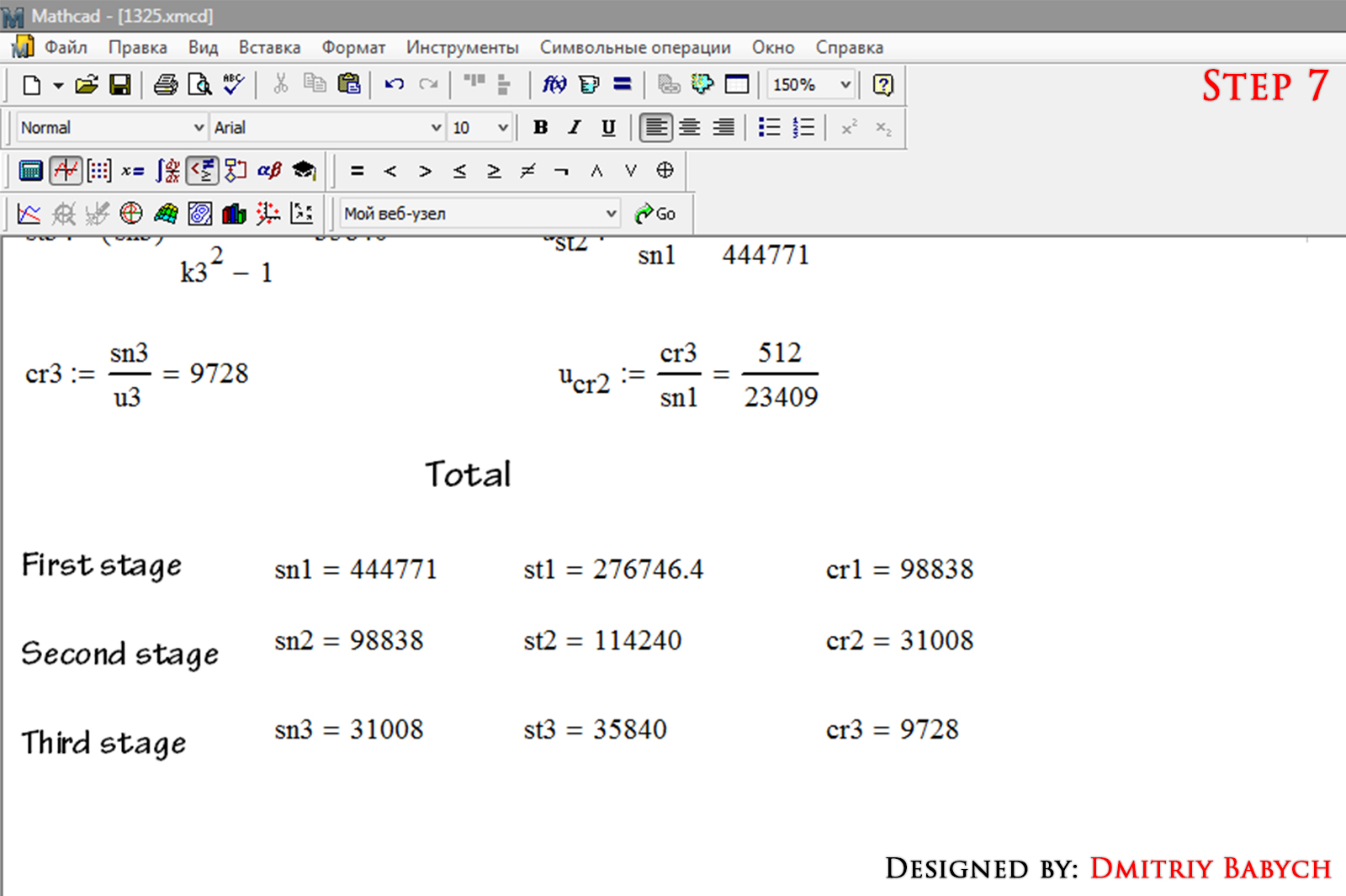8. ### Step 8:

Correct rotation of satellite from first stage. Divide the value by 2.4. We get 115311 degree. Its value matches the conditions from step 4. Now correct time of rotation. Divide the time value by 2.4 too. We get 5 min of rotation. This is enough to record animation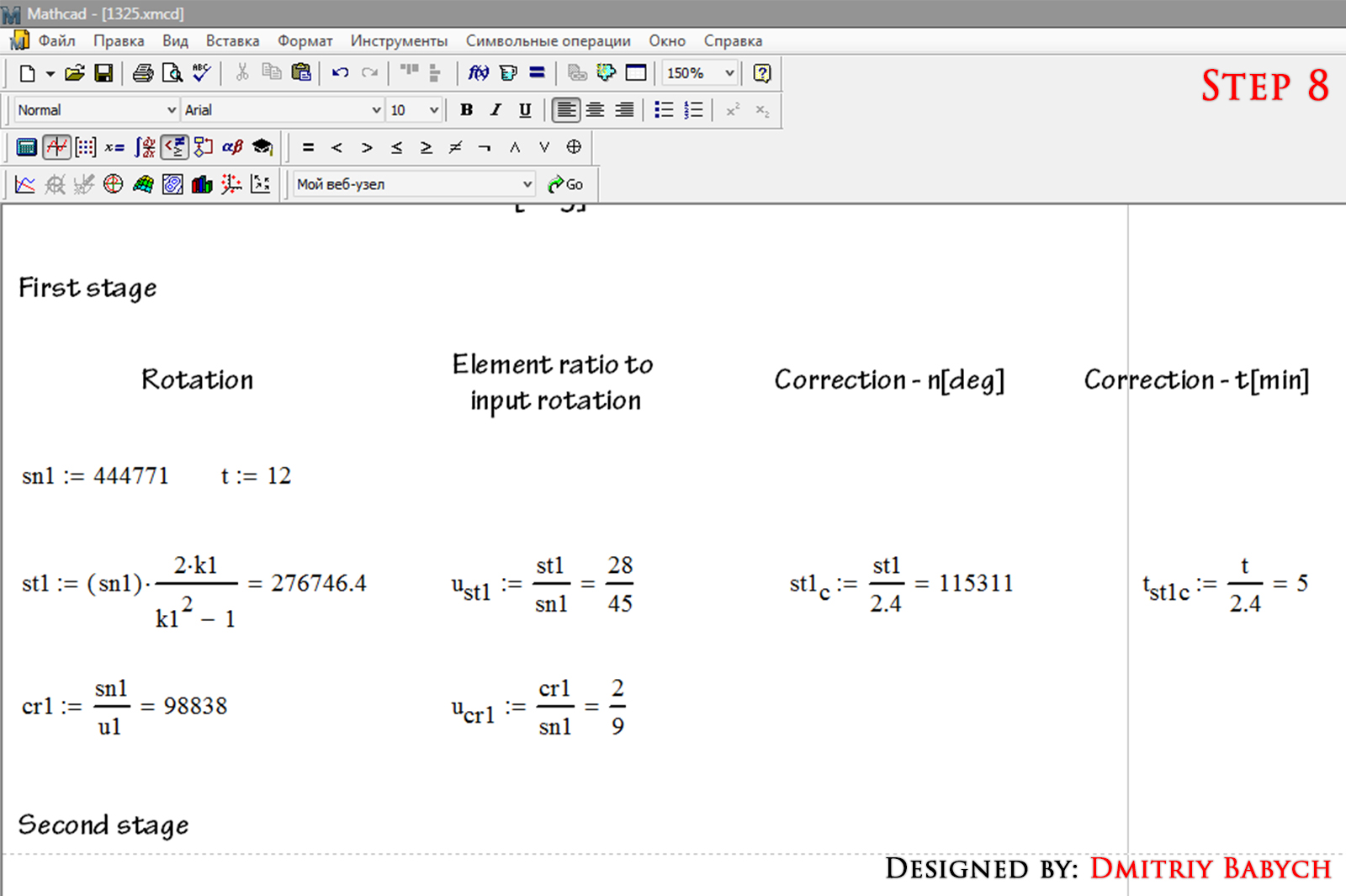9. ### Step 9:

To produce high-quality animation, we need to animate the bearings. We calculate rotation of the separator for each bearing. To simplify the calculation we will use in keyshot approximate values of rotation. Depending on movable bearing ring the formulas are different. All calculations in the screenshots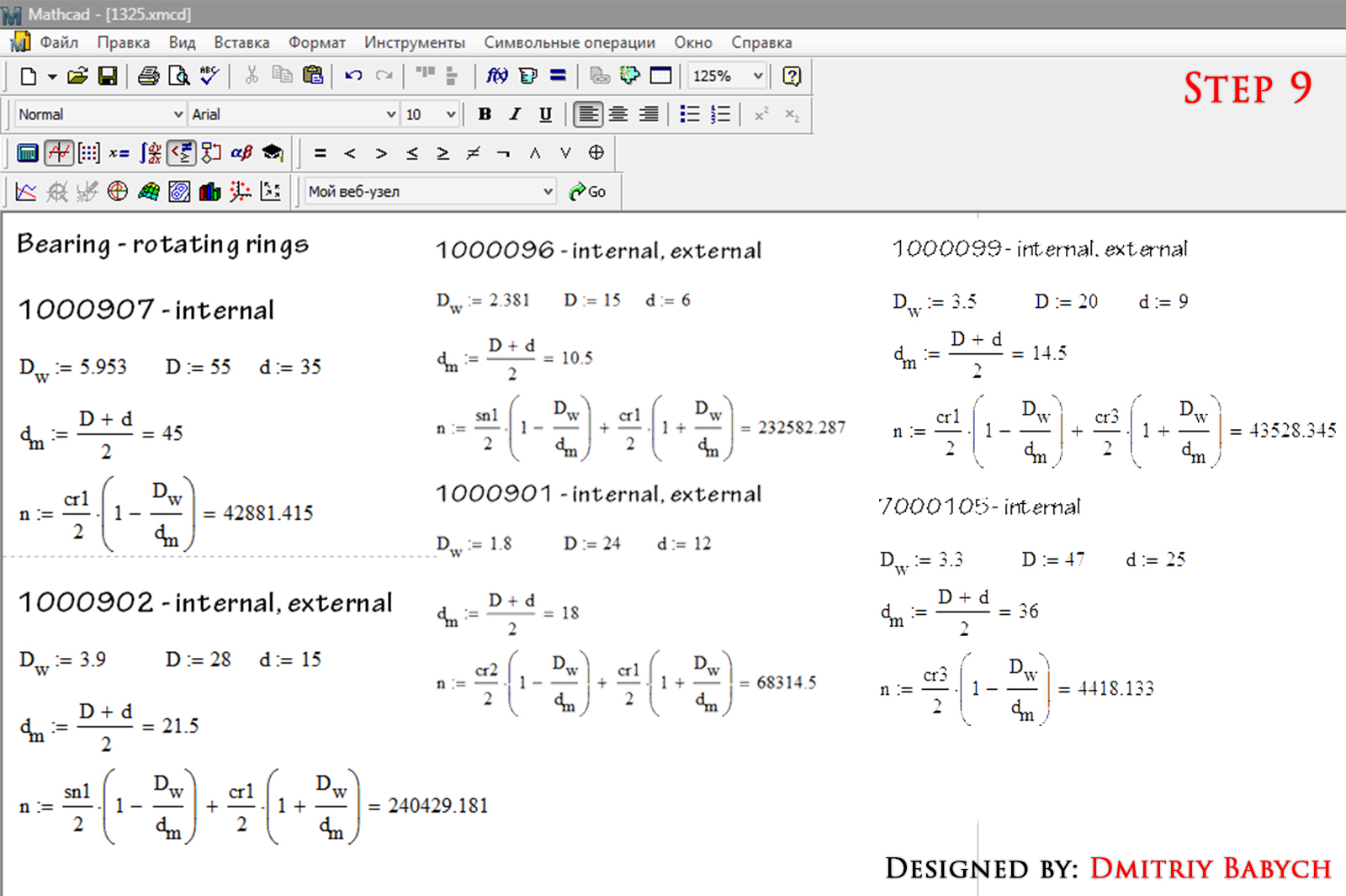10. ### Step 10:

Calculate rotation of 1000095 bearings, which used in satellites
Don’t forget correct rotation of bearing from first stage.
All calculations done! Congrats!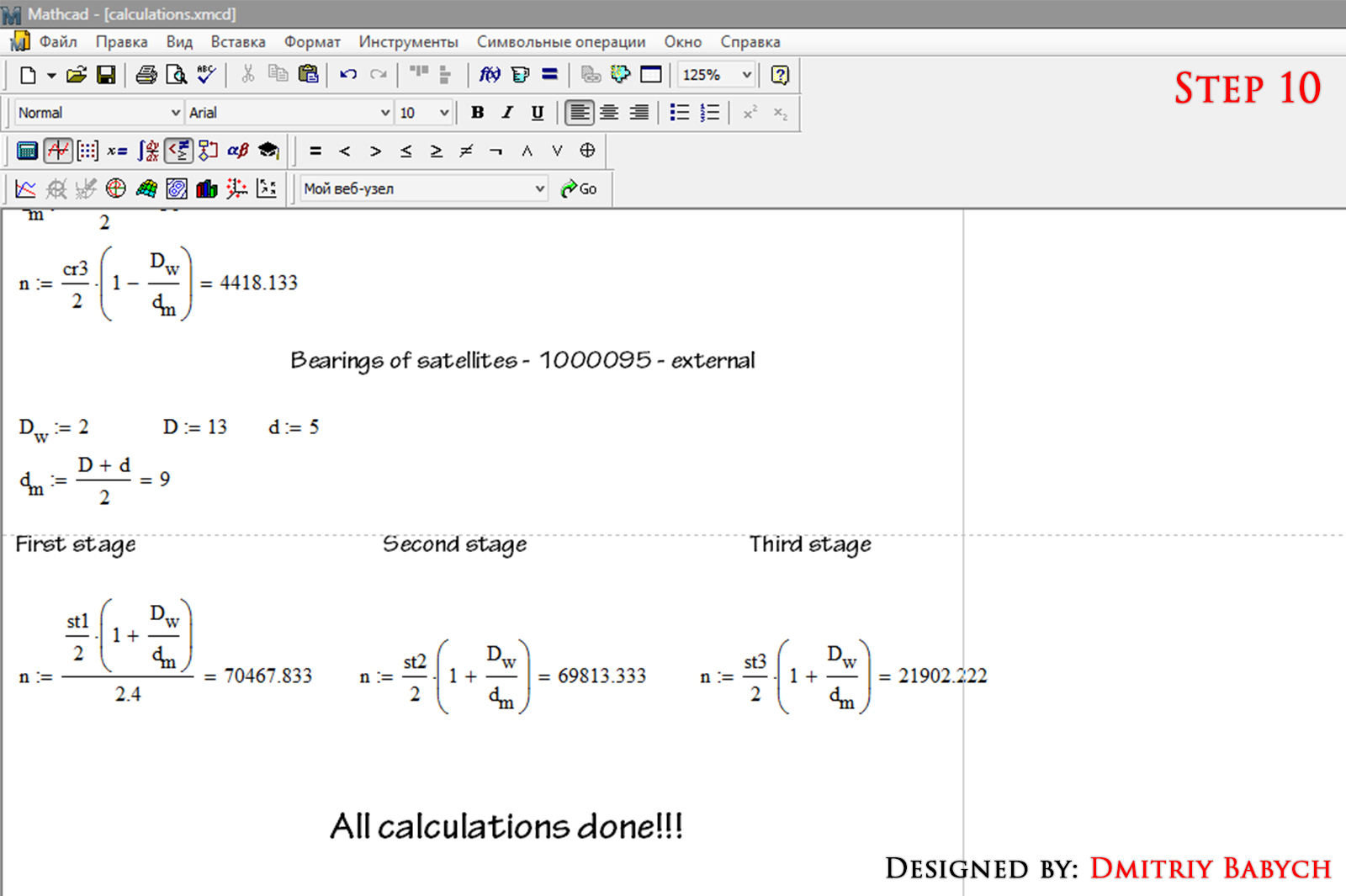11. ### Step 11:

Import model in Keyshot. Add materials, light etc. How it do you always can see in this tutorial https://grabcad.com/questions/tutorial-how-to-make-render-of-the-gearbox-in-keyshot-make-an-exploded-view-and-present-it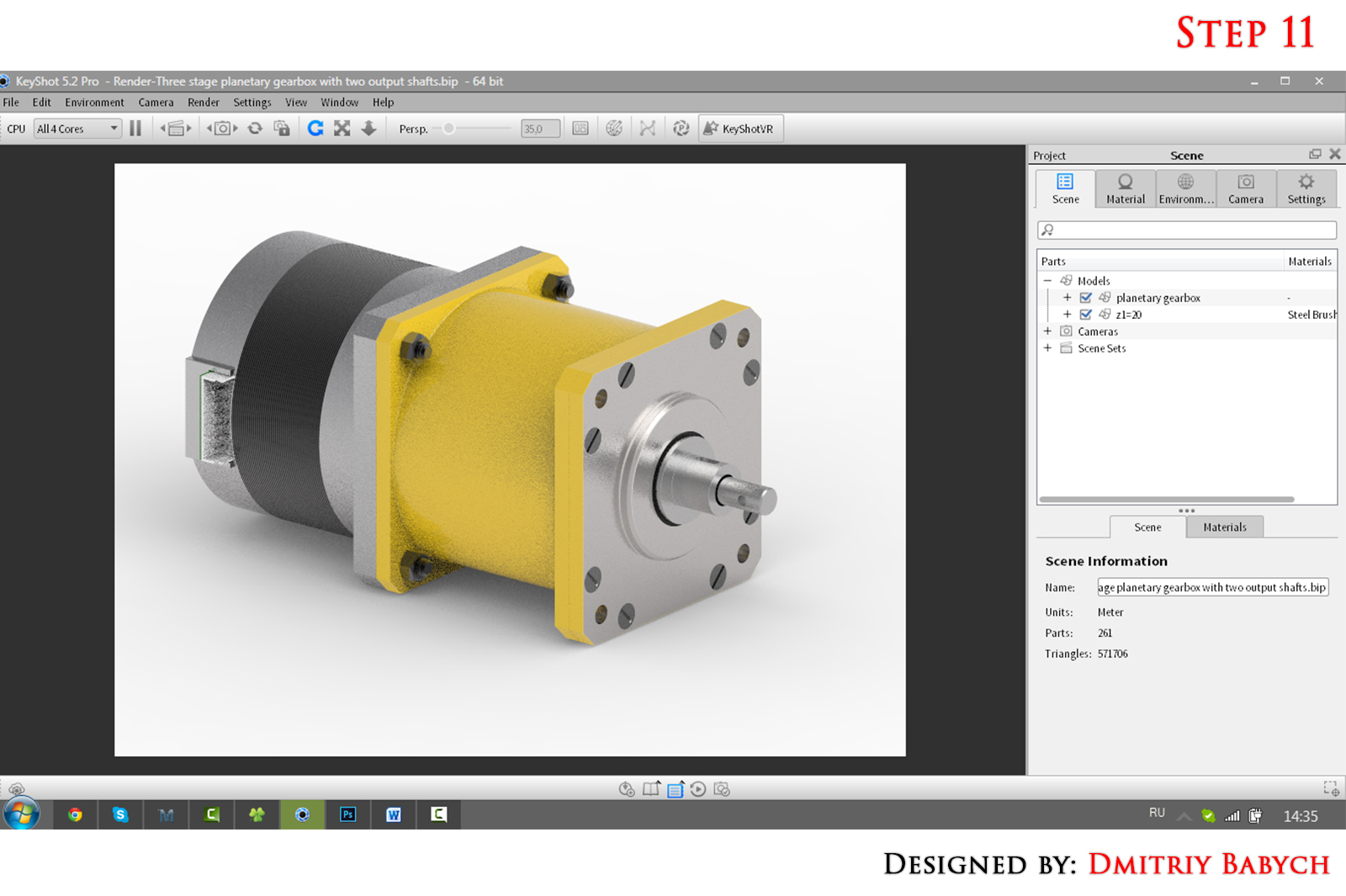12. ### Step 12:

Create a group of objects for which will be set animation.
For example, for first stage will be next group tree:
First stage
carrier
satellite 25 (include bearings 1000095 (include group with balls and separately two rings), axle, distance bushing) x3

How do groups I showed in this vid https://grabcad.com/questions/tutorial-how-to-make-render-of-the-gearbox-in-keyshot-make-an-exploded-view-and-present-it.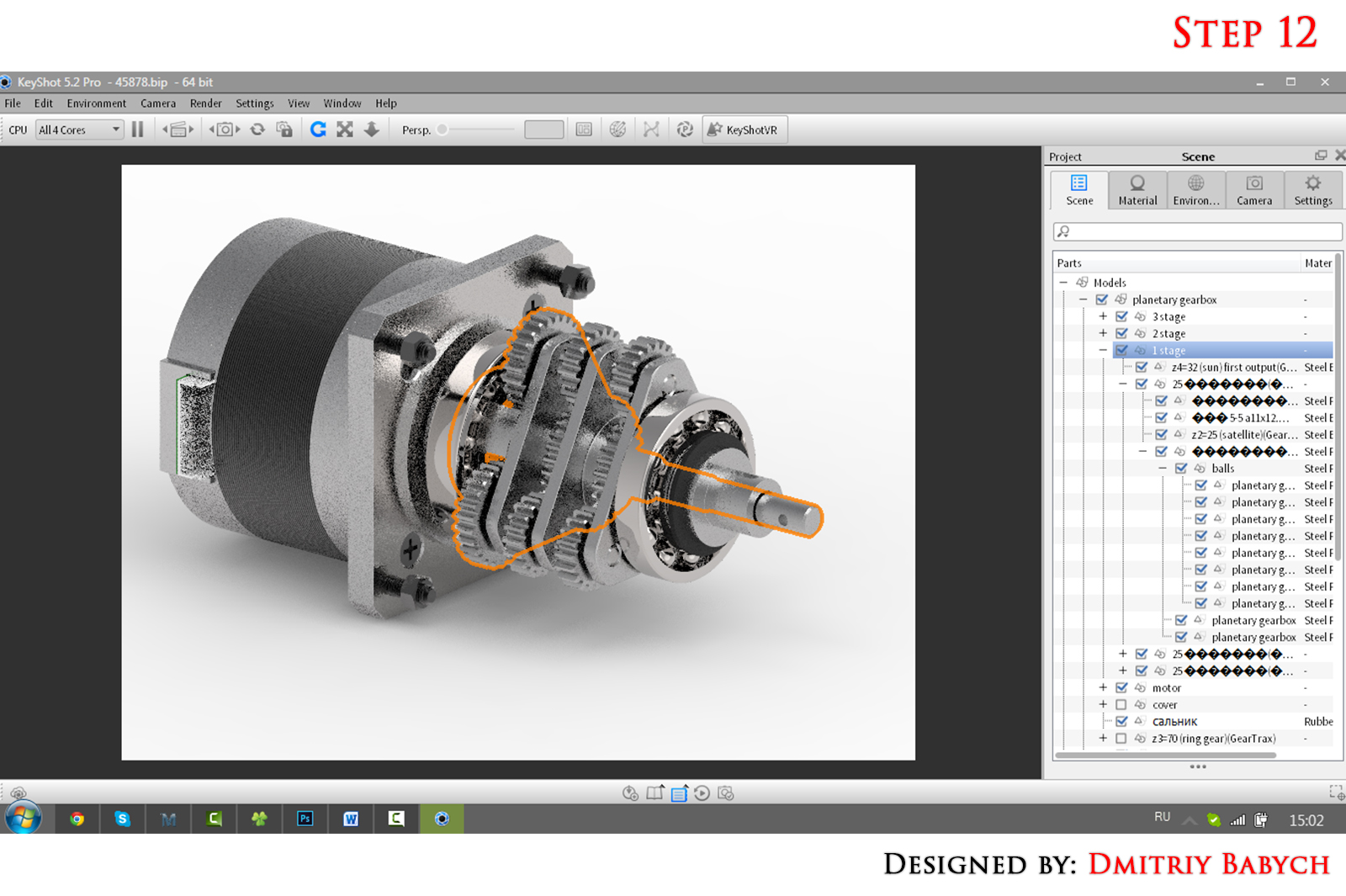13. ### Step 13:

Create groups with other parts. You need get next groups: 1st stage, 2st stage, 3th stage, motor, cover. For every bearing you must create group with balls.
Creating groups is one of the main parts of the work, be careful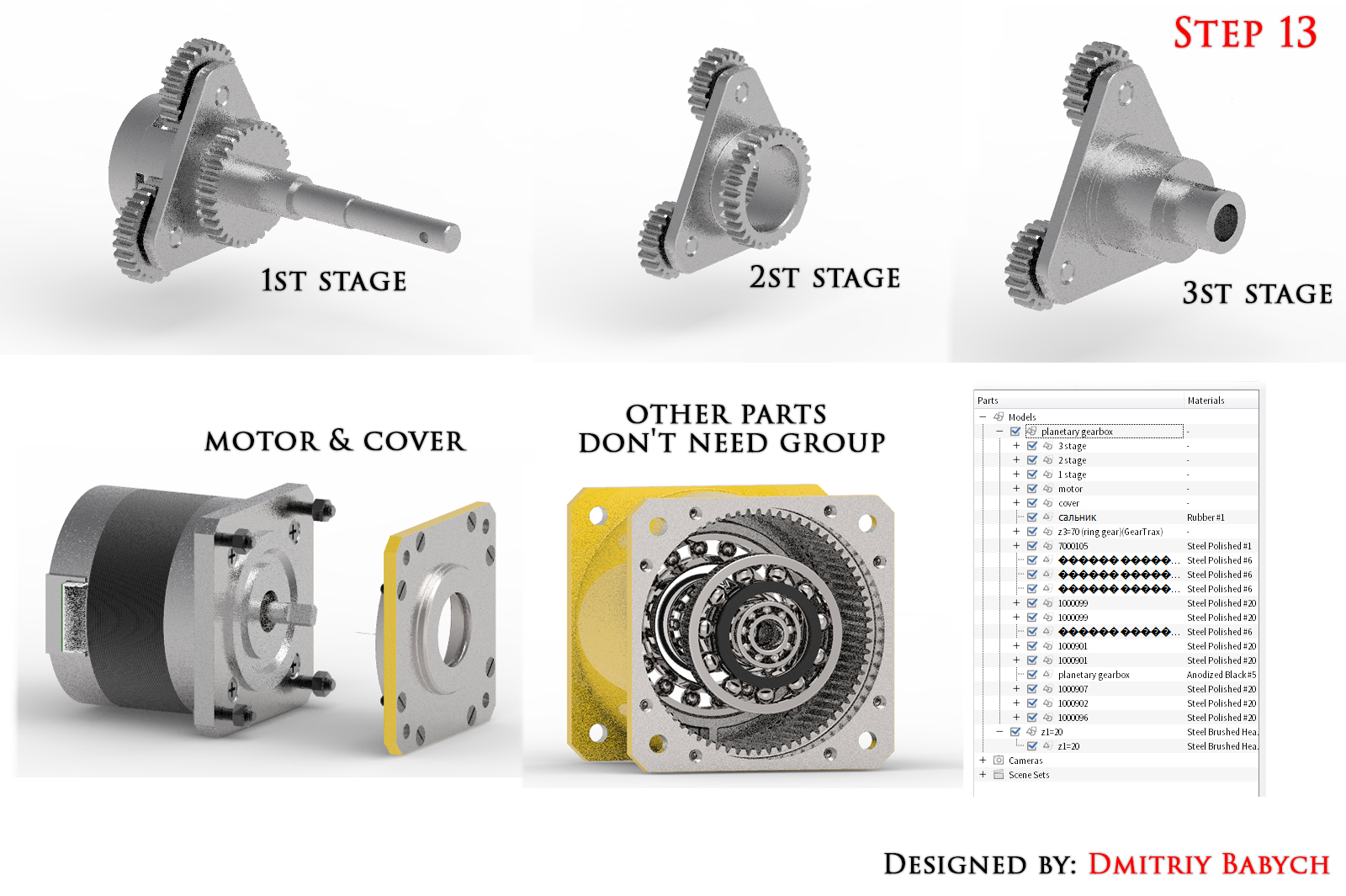14. ### Step 14:

Show only satellites from first stage, sun gear z=20 and body. Set orthographic front view.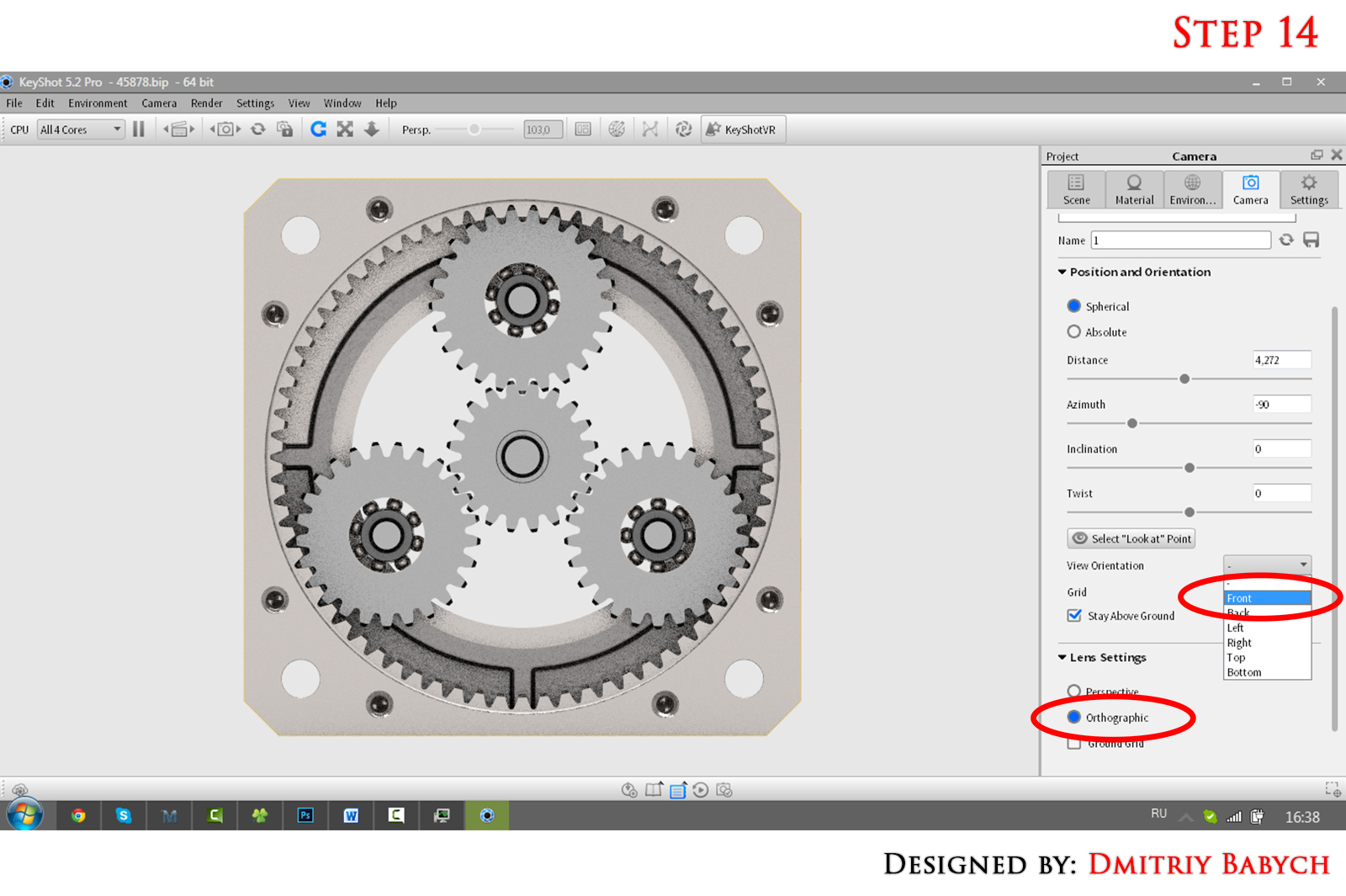15. ### Step 15:

Using calculations from step 1-10, set animation for sun gear: rotation 444771, axis Z, time start 0.00.00, time end 12.00.000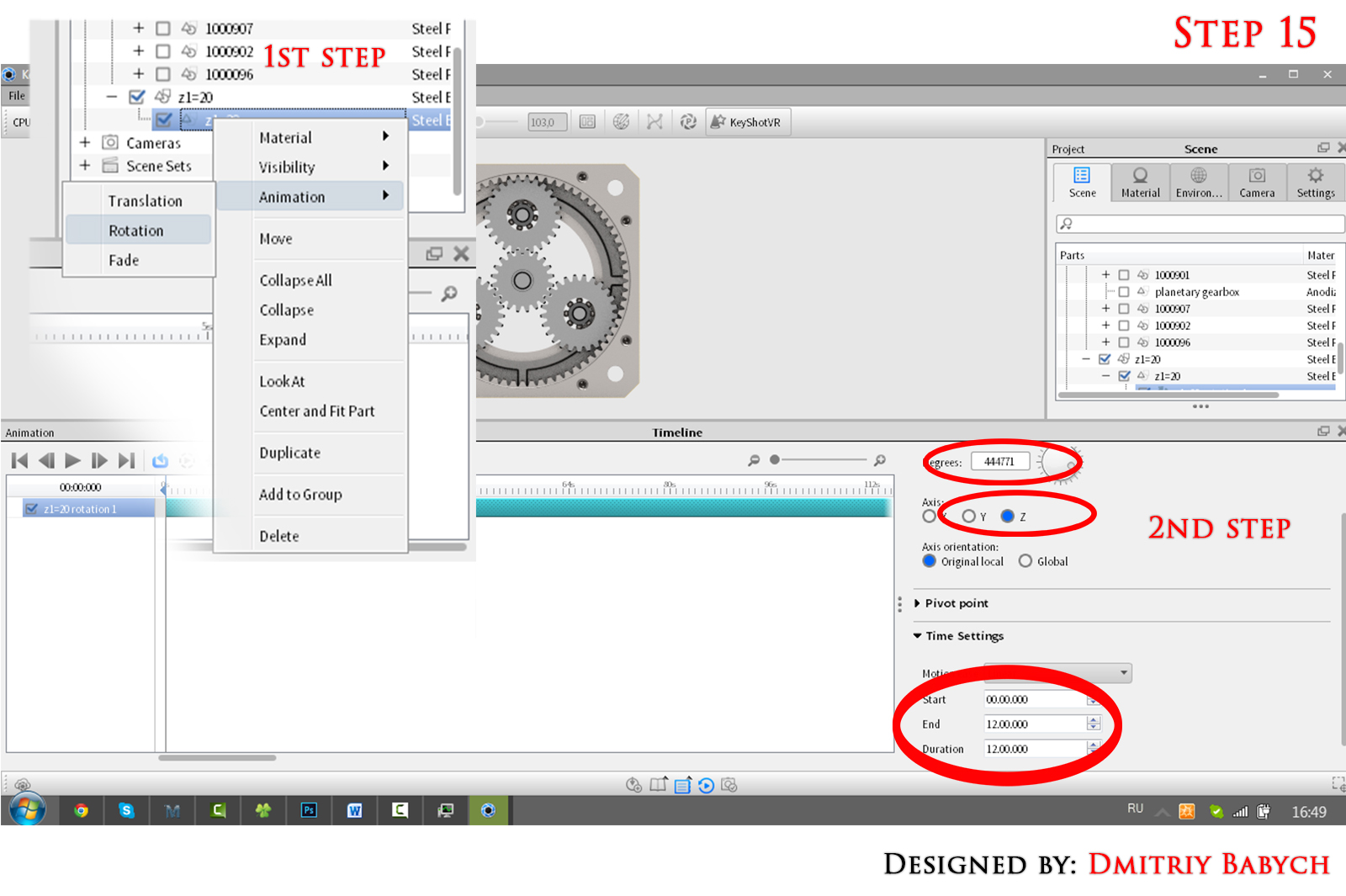16. ### Step 16:

Add animation to 1st stage. Set rotation -98838, axis Z, time start 0.00.00, time end 12.00.000
For every stage (1st, 2nd , 3th) you must select “origin pivot point”. Its nesesary!! If this does not make the item will be rotate around the center of its overall dimension, not to the shaft axis. In some detail axes pointing in different directions. Adjust the direction of rotation of the “–“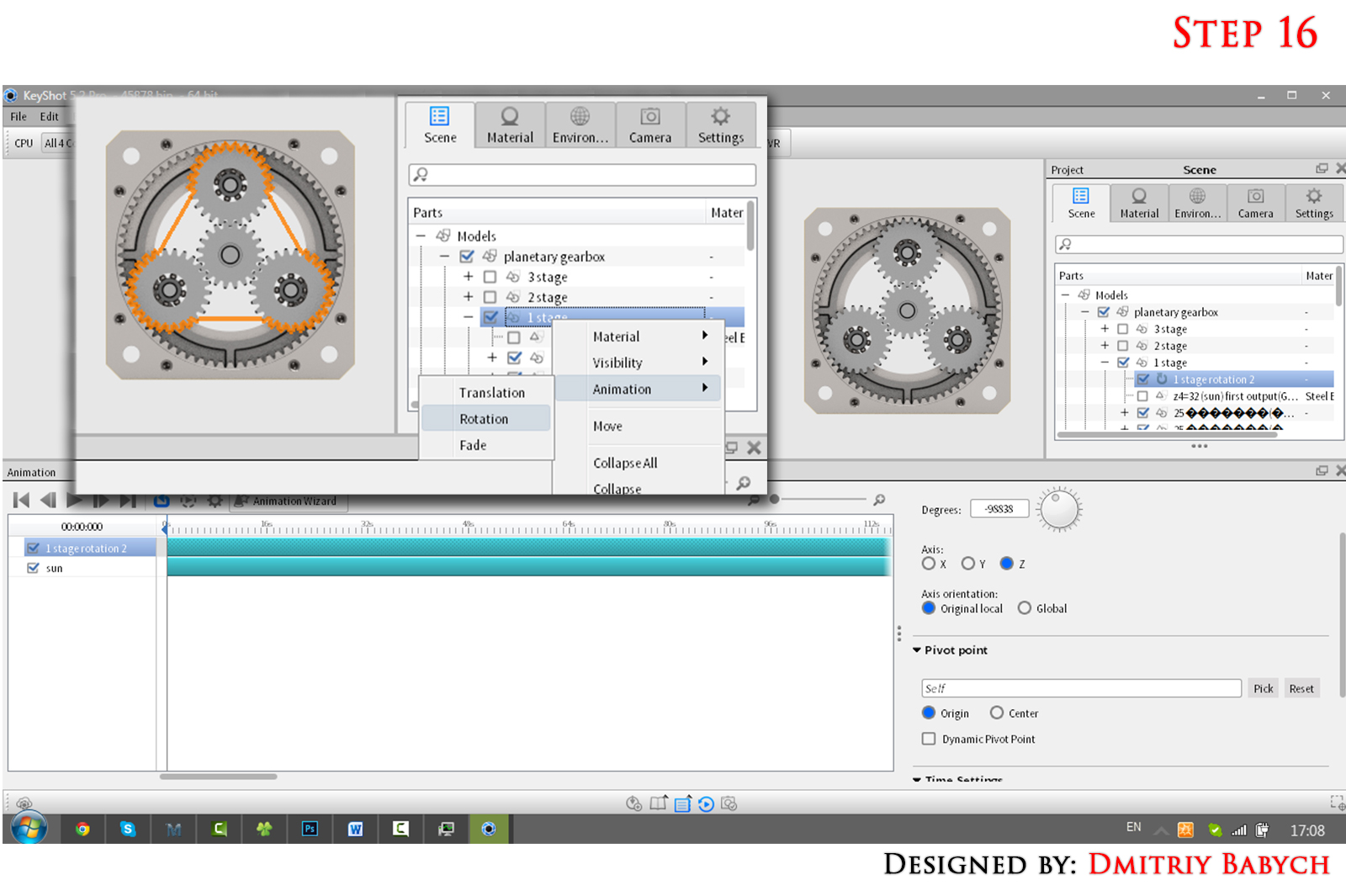17. ### Step 17:

Add animation to satellite. Set rotation -115311 axis Z. We corrected rotation of this element that why, set time start 0.00.00, time end 5.00.000. In all other cases (except bearing of those satellites) the duration of the rotation will be 12 minutes.
Copy and paste linked animation to other two satellites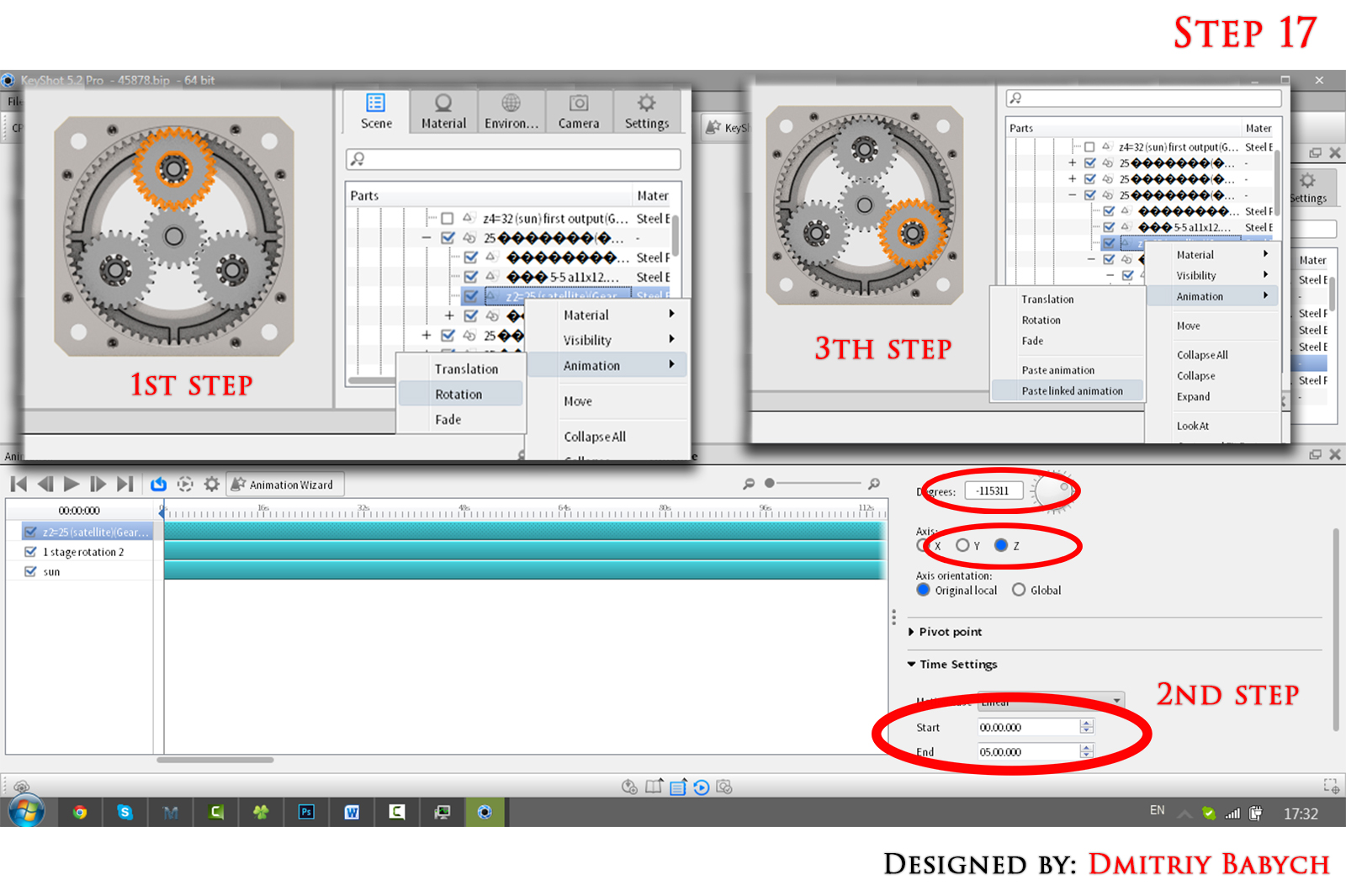18. ### Step 18:

Add animation to bearings of satellites. You must add rotation only for group with balls of bearing. Set rotation 70467, axis X, time start 0.00.00, time end 5.00.000
Copy and paste linked animation to other two bearings.
Animation of first stage is made!!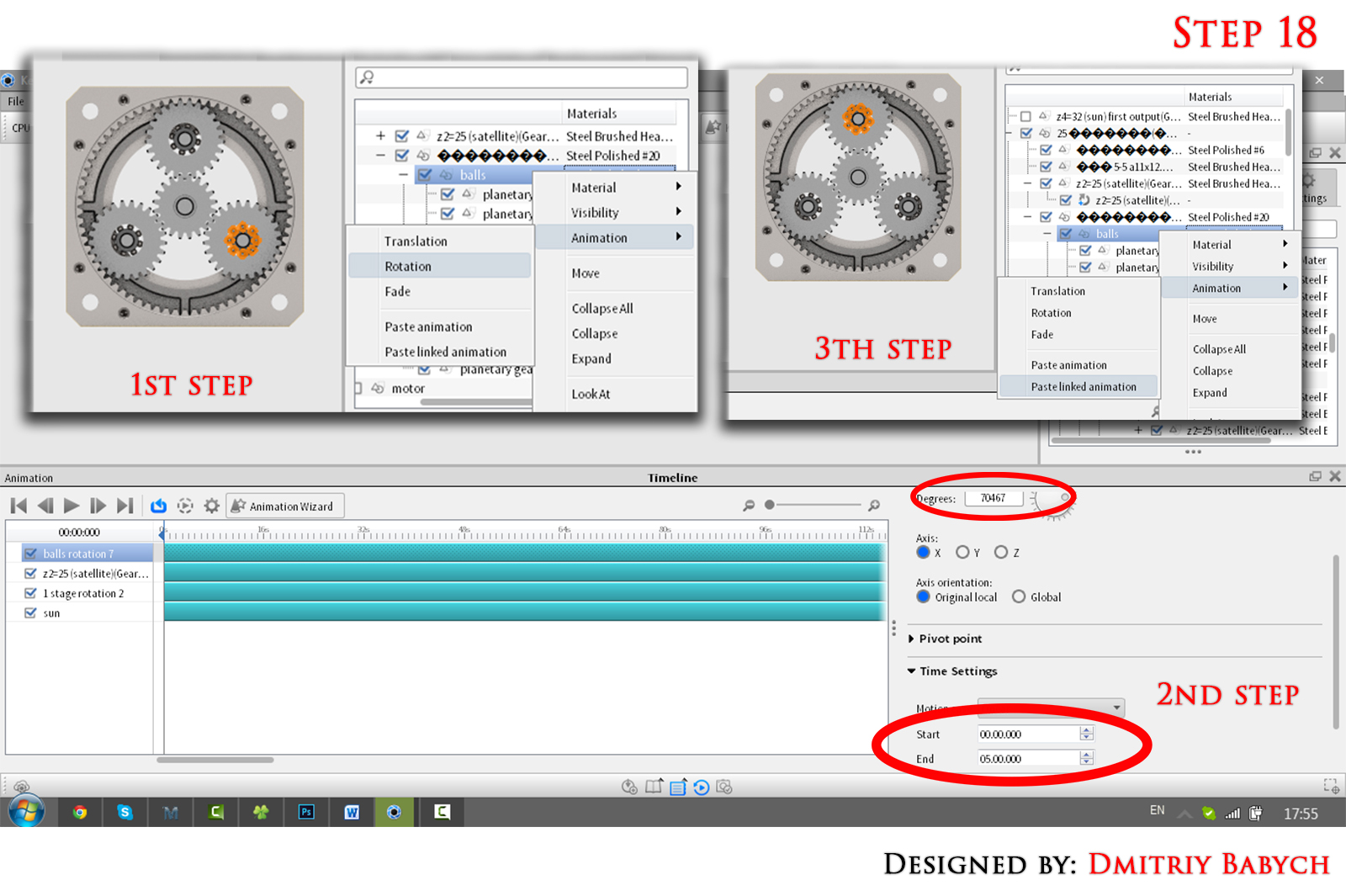19. ### Step 19:

Repeat steps 15-18 for all parts that rotate. Rotation Time will be the same for all - 12 minutes. Be careful with the direction of rotation. All steps must rotate in one direction, the satellites - in the other. Bearings are rotated into the same direction as the shaft on which they are located.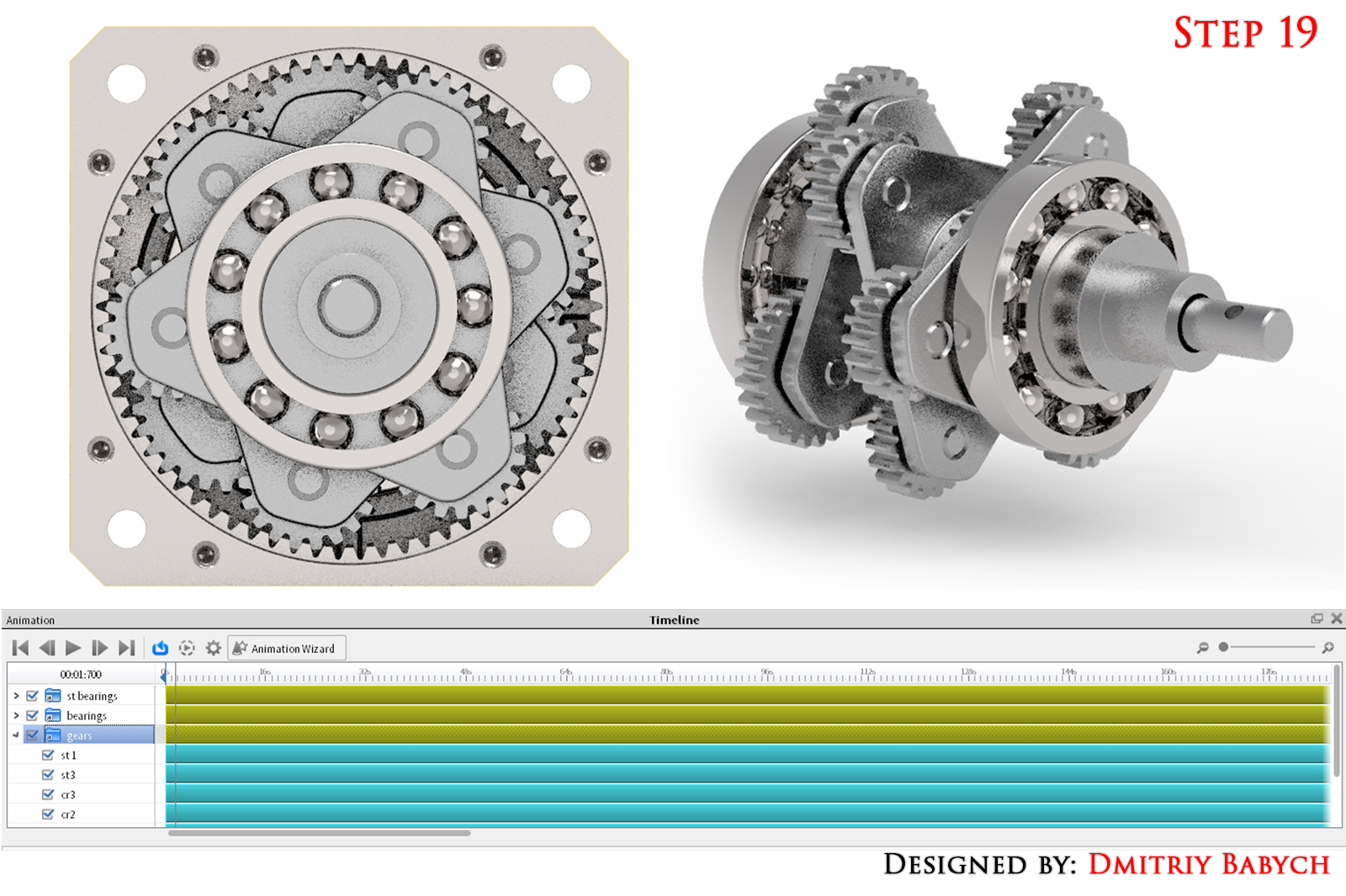20. ### Step 20: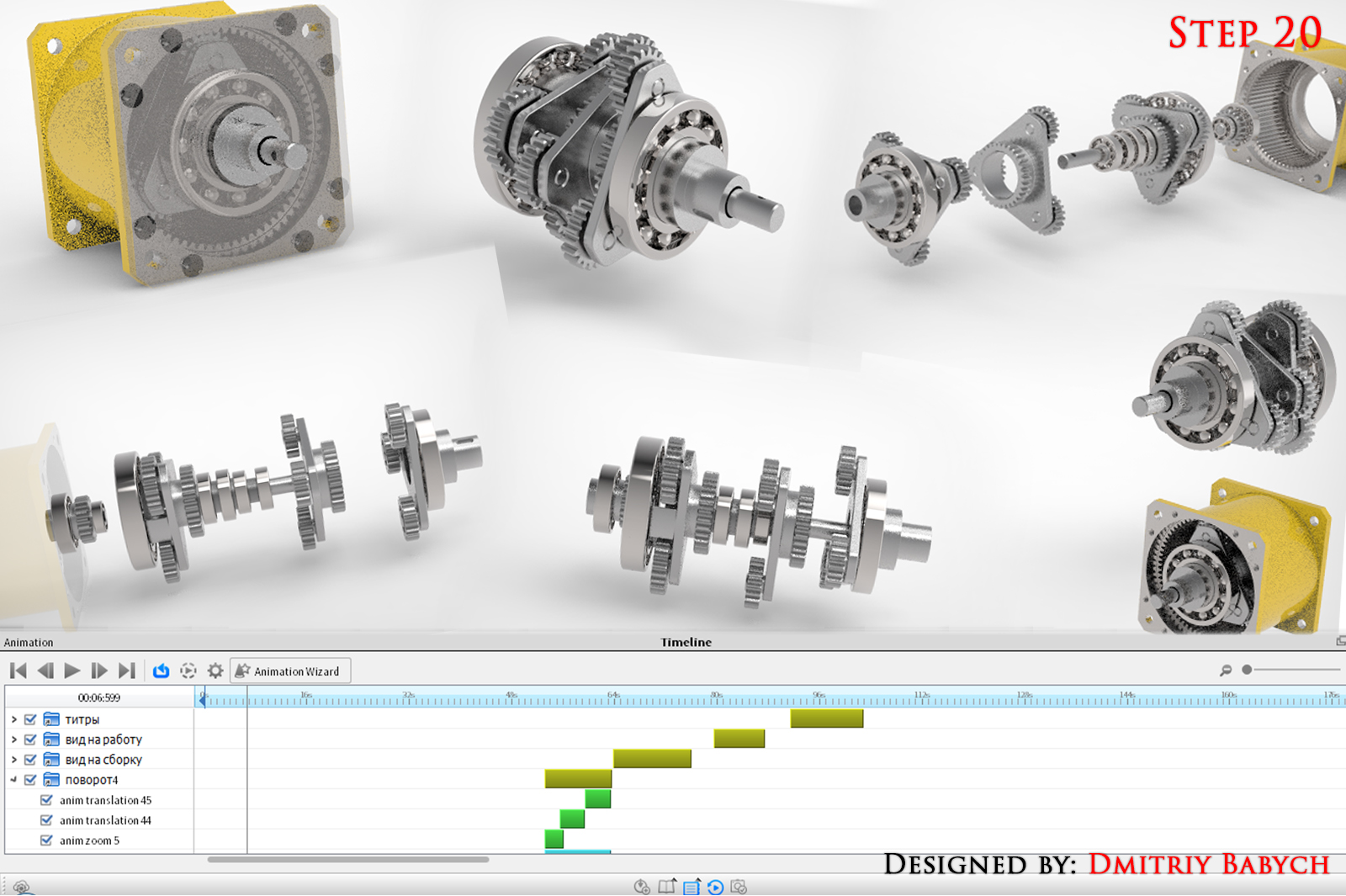21. ### Step 21:

Turn on the option of render: self-shadows (recommended), global illumination (recommended), ground illumination (optional), focused causing (optional).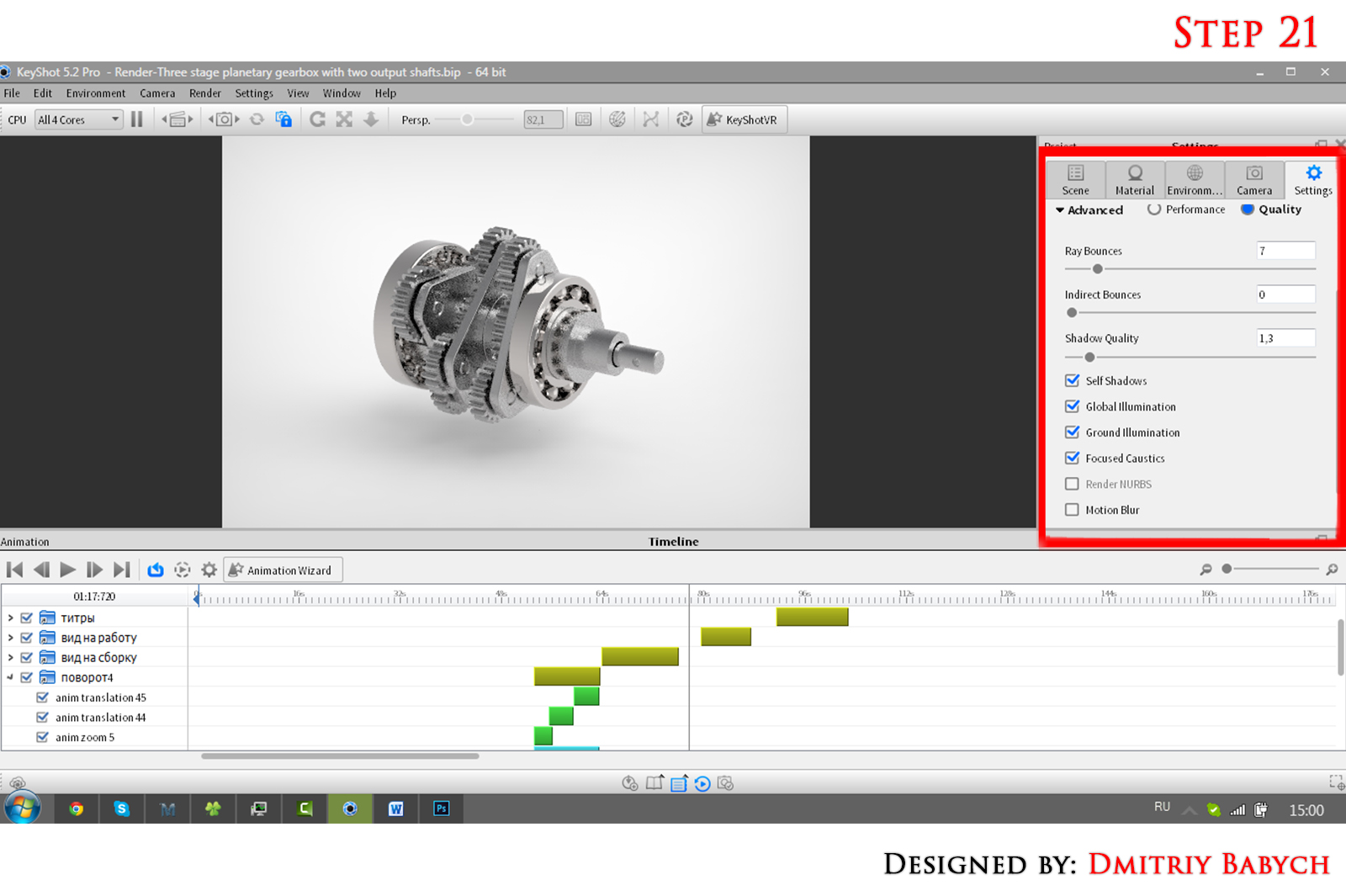22. ### Step 22:

Depending on the power of PC, set the parameters of the rendering and render it!
Good luck!!